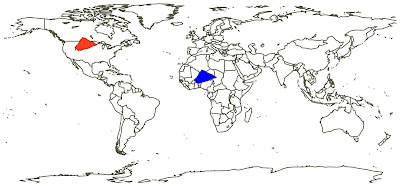## Saturday, December 13, 2008

### How big is your species-area polygon?

Following on from my dino world post I have recently been trying to work out how to calculate the area of a species' (or other taxon's) range given a set of occurrences as latitudes and longitudes. In theory this is very simple: you draw a convex hull around your occurrences, split the resulting polygon into triangles, measure the area of each and sum the results. Simple, right?

Trouble is, when your coordinates are actually latitudes and longitudes things aren't as easy. Imagine this simple scenario:Which polygon is largest, the blue or the red? On this projection they appear equal and this is because the distance, measured in degrees of latitude and longitude, between each set of points is exactly the same. However, although latitude is truly a measure of distance (one degree is 111km) longitude is not. At the equator one degree of longitude is equal in distance to one degree of latitude, but closer to the poles this distance declines to zero. This is because our polygon is really a spherical polygon.

How do we measure the area of a spherical polygon? Well, in the same way. we split it into spherical triangles and measure the area of these. However, to measure the area of a spherical triangle we need to know, not the lengths, but the angles (A, B and C, given in radians) as well as the radius (R) of our sphere:

So all we really need to do is work out those angles, but our data is latitudes and longitudes. More technically, we are providing the location of our polygon's vertices.

The easiest way to do this (Bevis and Cambareri 1987) is to artificially move each point to the pole, then the angle can be calculated as the difference between longitudes. However, this isn't quite as simple as it sounds as each angle can be measured twice, once for the 'interior' and once for the 'exterior'. So, for example, there may be 40 degrees of separation in one direction and 360 - 40 = 320 degrees in the other. The reason this confusion arises is that the sides of a spherical polygon are actually the sides of two spherical polygons, both an 'inside' and an 'outside'. (Both are finite, and hence, both are measurable).

Bevis and Cambareri (1987) suggest a way around this is to use an algorithm that measures the area based on the sequence the points are fed to it:If the points are fed in clockwise order then the internal angles, and hence internal polygon (1) is calculated (shaded area, left-hand plot). If in anticlockwise order then the external polygon (2) is measured (shaded area, right-hand plot).

So how big is your species-area polygon? Well, if you really want to know you can calculate it using the Bevis and Cambareri method (I have put an R implementation on my website here).

Reference:

Bevis, M. and G. Cambareri, 1987. Computing the area of a spherical polygon of arbitrary shape. Mathematical Geology, 19, 335-346.